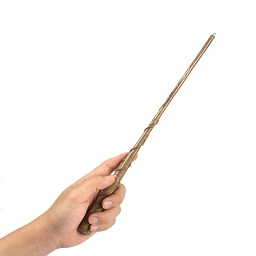### E - Elder Wand

##### Languages: C, C++, Java, Python, ... (details)

After having watched all eight Harry Potter movies in a week, Fito finally realized how the famous Elder Wand changes the wizard it obeys. If wizard A, whom the wand is currently obeying, is defeated by wizard B in a duel, then the wand will start obeying the wizard B.Fito is now wondering what would happen if $26$ wizards labelled with upper case letters of the English alphabet from "$A$" to "$Z$" began fighting in duels for the fondness of the Elder Wand. If we know the label of the wizard that the wand had obeyed before all duels and the outcomes of all $N$ duels that were held one after another, answer the following questions:

1. Which wizard did the wand obey after all $N$ duels?
2. How many different wizards did the wand obey?

#### Input

The first line contains an uppercase letter of the English alphabet, the label of the wizard that the wand obeyed at the beginning.

The second line contains an integer number $N$ $(1 \leq N \leq 100)$, the number of duels from the text of the task.

In the next $N$ rows there are two different uppercase letters of the English alphabet: $x_1$ and $x_2$ separated by a space, which means that the wizard with the label $x_1$ defeated the wizard with the label $x_2$ in the $i$-th duel.

#### Output

In the first line print an uppercase letter of the English alphabet, answer to the first question from the task description. In the second line print an integer number, answer to the second question from the task description

#### Sample test(s)

Input
A 3 B A C B D A
Output
C 3
Input
N 5 D A N B B A C D F A
Output
N 1
Input
X 4 A X B X X A D A
Output
X 2